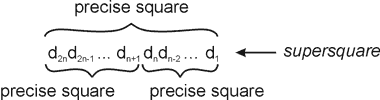Online JudgeProblem SetAuthorsOnline ContestsUser
Web Board
F.A.Qs
Statistical Charts
Problems
Submit Problem
Online Status
Prob.ID:
Register
Authors ranklist
Current Contest
Past Contests
Scheduled Contests
Award Contest
Register
Language:
Supersquare
 Time Limit: 1000MS Memory Limit: 65536K Total Submissions: 210 Accepted: 37 Special Judge

Description

Let number A be a precise square if there exists natural number B such that B*B = A.

2n-digit number without leading zeroes is called a supersquare if it is a precise square and both n-digit numbers which are formed from its n first digits and its n last digits are precise squares. The second n-digit number dndn-1…d1 formed from n last digits may have leading zeroes but must not be equal zero.You need to write a program which constructs a 2n-digit number which is supersquare.

Input

The input contains several test cases. The first line contains the number of test cases T (1 ≤ T ≤ 10). Each of the next T lines describes one test case and contains an integer number n (1 ≤ n ≤ 500).

Output

The output consists of T lines, one line per each test case. Each line contains 2n-digit supersquare number. If several solutions are possible only one of them should be given. When it is impossible to construct 2n-digit supersquare, the line must contain NO SUPERSQUARE POSSIBLE phrase.

Sample Input

```2
1
2```

Sample Output

```49
1681```

Source

Northeastern Europe 2005, Western Subregion

[Submit]   [Go Back]   [Status]   [Discuss]Home PageGo BackTo top